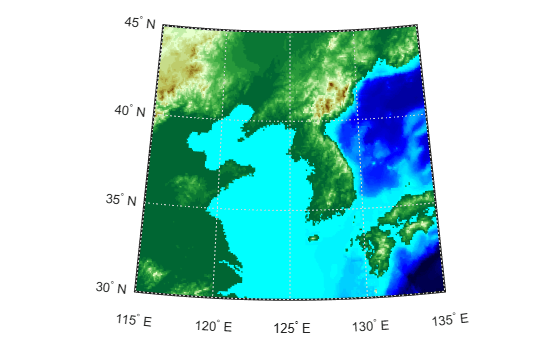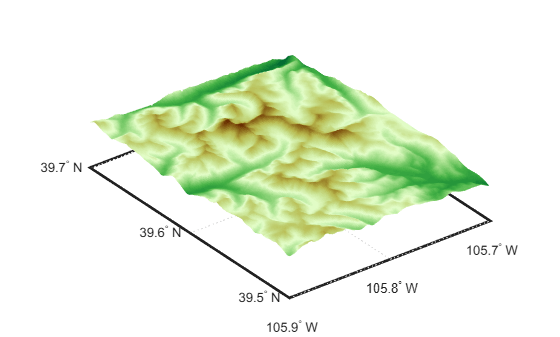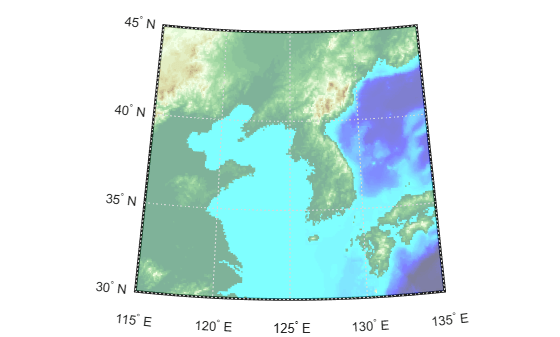# surfm

Project geolocated data grid on `axesm`-based map

## Syntax

``surfm(lat,lon,Z)``
``surfm(latlim,lonlim,Z)``
``surfm(lat,lon,Z,h)``
``surfm(___,Name,Value)``
``s = surfm(___)``

## Description

example

````surfm(lat,lon,Z)` displays a surface on the current `axesm`-based map by warping the geolocated data grid `Z`, with geographic coordinates `lat` and `lon`, to a projected graticule mesh. The color of the surface varies according to the values in `Z`. This syntax displays the surface in a horizontal plane with all height values set to 0.```
````surfm(latlim,lonlim,Z)` creates a graticule mesh using the latitude limits `latlim` and the longitude limits `lonlim`.```

example

````surfm(lat,lon,Z,h)` displays the surface using the heights specified by `h`.```

example

````surfm(___,Name,Value)` specifies surface properties using one or more name-value pair arguments. For example, `"FaceAlpha",0.5` creates a semitransparent plot.```
````s = surfm(___)` returns the primitive surface object. Use `s` to modify the plot after creation. For a list of properties, see Surface Properties.```

## Examples

collapse all

Load elevation data and a geographic cells reference object for the Korean peninsula. Extract the latitude and longitude coordinates from the reference object.

```load korea5c [lat,lon] = geographicGrid(korea5cR);```

Find the latitude and longitude limits of the data. Then, display the data on a world map using a colormap appropriate for elevation data.

```[latlim,lonlim] = geoquadline(lat,lon); worldmap(latlim,lonlim) surfm(latlim,lonlim,korea5c) demcmap(korea5c)```Read elevation data and a geographic postings reference object for a region around South Boulder Peak in Colorado. Crop the data to cover a smaller region. Extract the latitude and longitude coordinates from the reference object.

```[Z,R] = readgeoraster("n39_w106_3arc_v2.dt1","OutputType","double"); [Z,R] = geocrop(Z,R,[39.5 39.7],[-105.9 -105.7]); [lat,lon] = geographicGrid(R);```

Get the latitude and longitude limits of the data. Then, display the data as a surface by specifying the height data as the elevation data (`Z`). Apply a colormap appropriate for elevation data.

```[latlim,lonlim] = geoquadline(lat,lon); usamap(latlim,lonlim) surfm(lat,lon,Z,Z) demcmap(Z)```

View the region in 3-D.

`view(3)`Load elevation data and a geographic cells reference object for the Korean peninsula. Extract the latitudes and longitudes from the reference object.

```load korea5c [lat,lon] = geographicGrid(korea5cR);```

Find the latitude and longitude limits of the data. Then, display the data on a world map. Adjust the transparency of the surface plot using the `FaceAlpha` name-value argument.

```[latlim,lonlim] = geoquadline(lat,lon); worldmap(latlim,lonlim) surfm(lat,lon,korea5c,"FaceAlpha",0.5)```

Apply a colormap appropriate for elevation data.

`demcmap(korea5c)`## Input Arguments

collapse all

Latitude coordinates, specified as a vector or a matrix. The sizes of `lat` and `lon` must match.

• When `lat` and `lon` are matrices of the same size as `Z`, the `surfm` function displays each element of `Z` at the location specified by the corresponding elements of `lat` and `lon`.

• When `lat` and `lon` are matrices of a different size than `Z`, the `surfm` function creates a graticule mesh of the same size as `lat`.

• When `lat` and `lon` are vectors, the `surfm` function creates a graticule mesh of size `length(lat)`-by-`length(lon)` by replicating `lat` and `lon`.

Data Types: `double`

Longitude coordinates, specified as a vector or a matrix. The sizes of `lon` and `lat` must match.

• When `lat` and `lon` are matrices of the same size as `Z`, the `surfm` function displays each element of `Z` at the location specified by the corresponding elements of `lat` and `lon`.

• When `lat` and `lon` are matrices of a different size than `Z`, the `surfm` function creates a graticule mesh of the same size as `lat`.

• When `lat` and `lon` are vectors, the `surfm` function creates a graticule mesh of size `length(lat)`-by-`length(lon)` by replicating `lat` and `lon`.

Data Types: `double`

Geolocated data grid, specified as an m-by-n array.

Latitude limits for `Z`, specified as a two-element vector of the form `[latmin latmax]`, where `latmax` is greater than `latmin`.

The `surfm` function uses `latlim` and `lonlim` to create a graticule mesh of the same size as `Z`.

Data Types: `double`

Latitude limits for `Z`, specified as a two-element vector of the form `[lonmin lonmax]`, where `lonmax` is greater than `lonmin`.

The `surfm` function uses `latlim` and `lonlim` to create a graticule mesh of the same size as `Z`.

Data Types: `double`

Surface heights, specified as a scalar or a matrix.

• When you specify a scalar, the `surfm` function displays the grid in the horizontal plane with all height values set to `h`.

• When you specify a matrix, the `surfm` function displays each vertex of the graticule mesh at the corresponding height in `h`. The size of the matrix must match the size of the graticule mesh.

When the `MapProjection` property of the `axesm`-based map is `"globe"`, `h` is referenced to the ellipsoid.

Data Types: `double`

### Name-Value Arguments

Specify optional pairs of arguments as `Name1=Value1,...,NameN=ValueN`, where `Name` is the argument name and `Value` is the corresponding value. Name-value arguments must appear after other arguments, but the order of the pairs does not matter.

Example: `surfm(lat,lon,Z,LineStyle="-")` displays the graticule mesh using a solid line.

Before R2021a, use commas to separate each name and value, and enclose `Name` in quotes.

Example: `surfm(lat,lon,Z,"LineStyle","-")` displays the graticule mesh using a solid line.

Note

Use name-value arguments to specify values for the properties of the `Surface` object created by this function. The properties listed here are only a subset. For a full list, see Surface Properties.

`Surface` objects created by the `surfm` function have these limitations:

• Setting the `XData`, `YData`, and `ZData` properties of the surface object is not supported.

• If you set the `UserData` property of the surface and then change the projection of the `axesm`-based map, MATLAB® does not reproject the surface.

Line style, specified as one of the options listed in this table.

Line StyleDescriptionResulting Line
`"-"`Solid line`"--"`Dashed line`":"`Dotted line`"-."`Dash-dotted line`"none"`No lineNo line

Line width, specified as a positive value in points, where 1 point = 1/72 of an inch. If the line has markers, then the line width also affects the marker edges.

The line width cannot be thinner than the width of a pixel. If you set the line width to a value that is less than the width of a pixel on your system, the line displays as one pixel wide.

## Output Arguments

collapse all

Primitive surface object, returned as a `Surface` object. Use `s` to modify the `Surface` object after creation. For a list of properties, see Surface Properties.

`Surface` objects created by the `surfm` function have these limitations:

• Setting the `XData`, `YData`, and `ZData` properties of the surface object is not supported.

• If you set the `UserData` property of the surface and then change the projection of the `axesm`-based map, MATLAB does not reproject the surface.

## Tips

• The `FaceColor` property of the surface object depends on the size of the graticule mesh. The `surfm` function sets `FaceColor` to `"flat"` when the size of the graticule mesh matches the size of `Z`, and `"texturemap"` otherwise.

• The `surfm` function projects the graticule mesh using the projection specified by the `MapProjection` property of the `axesm`-based map. To improve the resolution of the plot, increase the size of the graticule mesh. Increasing the size of the graticule mesh can increase the amount of time MATLAB requires to display the surface.

## Version History

Introduced before R2006a